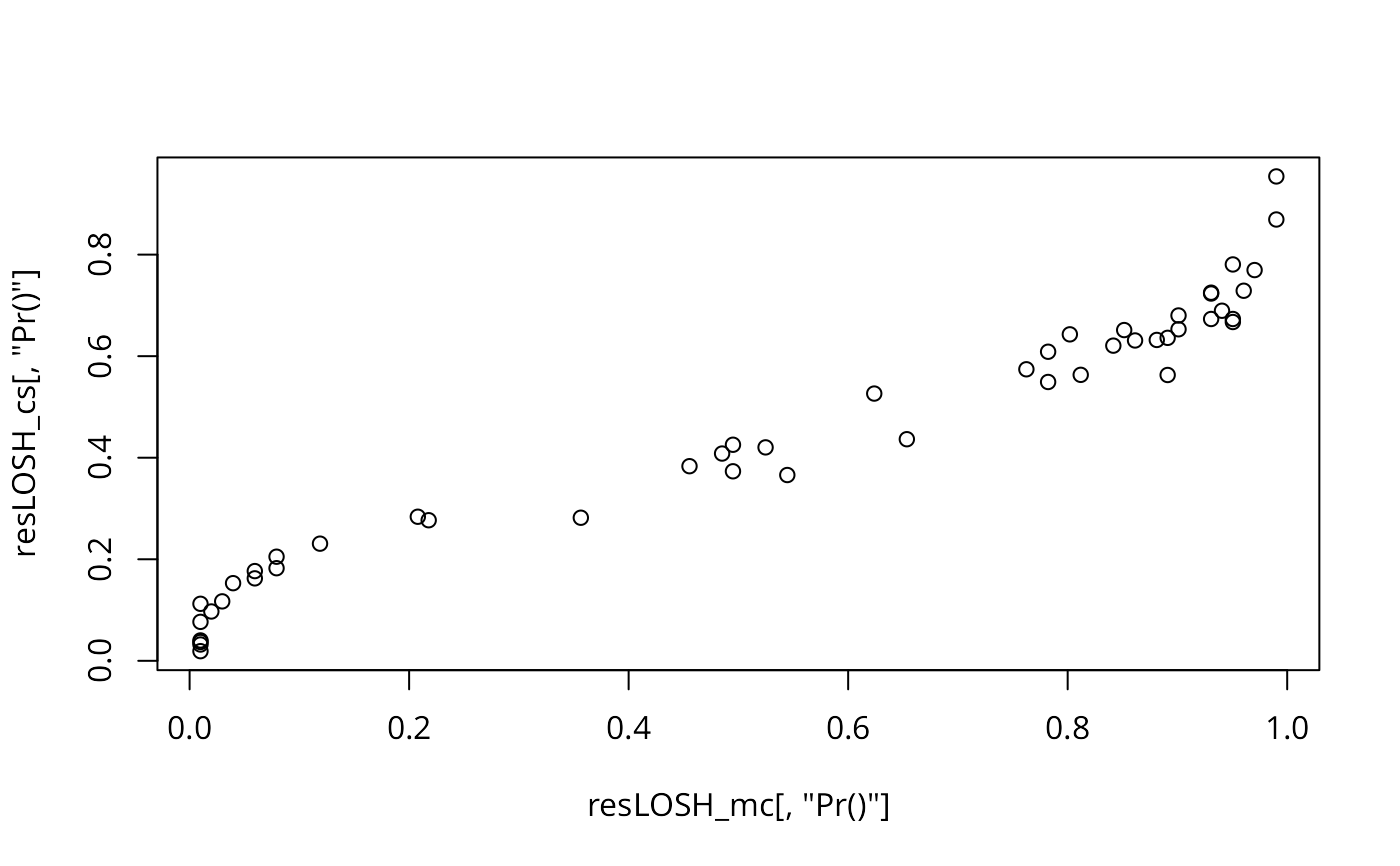The function draws inferences about local spatial heteroscedasticity (LOSH) by means of the randomisation-based Monte-Carlo bootstrap proposed by Xu et al. (2014).

LOSH.mc(x, listw, a = 2, nsim = 99, zero.policy = attr(listw, "zero.policy"),
na.action = na.fail, spChk = NULL, adjust.n = TRUE, p.adjust.method = "none")

## Arguments

x

a numeric vector of the same length as the neighbours list in listw

listw

a listw object created for example by nb2listw

a

the exponent applied to the local residuals; the default value of 2 leads to a measure of heterogeneity in the spatial variance

nsim

the number of randomisations used in the bootstrap

zero.policy

default attr(listw, "zero.policy") as set when listw was created, if attribute not set, use global option value; if TRUE assign zero to the lagged value of zones without neighbours, if FALSE assign NA

na.action

a function (default na.fail), can also be na.omit or na.exclude - in these cases the weights list will be subsetted to remove NAs in the data. It may be necessary to set zero.policy to TRUE because this subsetting may create no-neighbour observations. Note that only weights lists created without using the glist argument to nb2listw may be subsetted. If na.pass is used, zero is substituted for NA values in calculating the spatial lag. (Note that na.exclude will only work properly starting from R 1.9.0, na.omit and na.exclude assign the wrong classes in 1.8.*)

spChk

should the data vector names be checked against the spatial objects for identity integrity, TRUE, or FALSE, default NULL to use get.spChkOption()

default TRUE, if FALSE the number of observations is not adjusted for no-neighbour observations, if TRUE, the number of observations is adjusted

a character string specifying the probability value adjustment for multiple tests, default "none"; see p.adjustSP. Note that the number of multiple tests for each region is only taken as the number of neighbours + 1 for each region, rather than the total number of regions.

## Details

The test calculates LOSH (see LOSH) and estimates pseudo p-values from a conditional bootstrap. Thereby, the i-th value in each location is held fixed, whereas all other values are permuted nsim times over all other spatial units.

## Value

Hi

LOSH statistic

E.Hi

expectation of LOSH

Var.Hi

variance of LOSH

Z.Hi

the approximately chi-square distributed test statistics

x_bar_i

local spatially weighted mean values

ei

residuals about local spatially weighted mean values

Pr()

p-values for Hi obtained from a conditional bootstrap distribution

## Author

René Westerholt rene.westerholt@tu-dortmund.de

LOSH, LOSH.mc

## Examples

    data(columbus, package="spData")
resLOSH_mc <- LOSH.mc(columbus$CRIME, nb2listw(col.gal.nb), 2, 100) summary(resLOSH_mc) #> Hi x_bar_i ei Pr() #> Min. :0.03438 Min. :13.85 Min. : 0.0298 Min. :0.009901 #> 1st Qu.:0.23838 1st Qu.:24.71 1st Qu.: 7.0114 1st Qu.:0.148515 #> Median :0.66689 Median :35.90 Median : 52.1094 Median :0.673267 #> Mean :1.06592 Mean :34.88 Mean : 151.9232 Mean :0.550212 #> 3rd Qu.:1.59680 3rd Qu.:45.39 3rd Qu.: 105.0551 3rd Qu.:0.891089 #> Max. :4.68765 Max. :54.91 Max. :2455.2201 Max. :0.990099 resLOSH_cs <- LOSH.cs(columbus$CRIME, nb2listw(col.gal.nb))
summary(resLOSH_cs)
#>        Hi               E.Hi       Var.Hi            Z.Hi
#>  Min.   :0.03438   Min.   :1   Min.   :0.4972   Min.   :0.04356
#>  1st Qu.:0.23838   1st Qu.:1   1st Qu.:0.9136   1st Qu.:0.35069
#>  Median :0.66689   Median :1   Median :1.4342   Median :1.19175
#>  Mean   :1.06592   Mean   :1   Mean   :1.4758   Mean   :1.89447
#>  3rd Qu.:1.59680   3rd Qu.:1   3rd Qu.:1.9547   3rd Qu.:2.65120
#>  Max.   :4.68765   Max.   :1   Max.   :2.9958   Max.   :7.25681
#>     x_bar_i            ei                 Pr()
#>  Min.   :13.85   Min.   :   0.0298   Min.   :0.0190
#>  1st Qu.:24.71   1st Qu.:   7.0114   1st Qu.:0.2050
#>  Median :35.90   Median :  52.1094   Median :0.5264
#>  Mean   :34.88   Mean   : 151.9232   Mean   :0.4507
#>  3rd Qu.:45.39   3rd Qu.: 105.0551   3rd Qu.:0.6529
#>  Max.   :54.91   Max.   :2455.2201   Max.   :0.9540
plot(resLOSH_mc[,"Pr()"], resLOSH_cs[,"Pr()"])